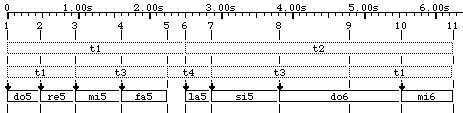9. Make it swing with smooth time...

So far we played around with musical items that were set on striated time, i.e. with a regular beat imposed by the time base (metronome, see §4.3). Indeed it was possible to change the metronome setting (although not in the middle of an item) and the time setting algorithm provided for infinite flexibility with sound-objects relocated or truncated on the basis of individual properties. However it seemed impossible to program an accelerando or some non-metric time-pattern like the typical elongated third beat in Saturday evening's waltz.

With smooth time you will be able to combine "plasticity" with accuracy. Suppose for instance that you want BP2 to play

do5 re5 mi5 fa5 - la5 si5 do6_ mi6
(in which '-' is a silence and '_' is the prolongation of do6) with the following time-pattern:Fig.27 Time-pattern with irregular beats

"t1", "t2", etc. are time-objects, somehow similar to sound-objects except that they do not contain any sound-generating code. Here the piece is divided in two sections, namely "t1" and "t2", each of them lasting five beats with different physical durations . Let us assume that "t1" is a "normal duration" yielding five beats in 2.5 seconds, therefore we will set mm = 120. Here the metronome does not construct a regular pattern of time streaks. It only serves as a time reference. This case is referred as measured smooth time. The second section "t2" is also five beats but it lasts 3.75 seconds, i.e. 1.5 times the first section. When specifying time-patterns each duration will be written as an integer ratio. We will therefore write:

t1 = 1/1 t2 = 3/2
(We could write t2 = 150/100 or any equivalent ratio as well.) This statement contains three pieces of information: (1) t2 is 1.5 times longer than t1, (2) the default duration of t1 is one metronome beat, (3) the default duration of t2 is 3/2 metronome beats. Of course, (3) may be deducted from (1) and (2).

Let us now look at subdivisions. "t1" contains three subdivisions labelled "t1", "t3" and "t4". Of course, the new "t1" has been resized so that the three subdivisions fit exactly in the first section. Only ratios are important at this stage. You may measure that t3 is 1.33 times longer than t1, and t4 is two times shorter. Therefore we will write

t3 = 4/3 t4 = 1/2
(or equivalent integer ratios). The second section is subdivided as "t3" and "t1" in which the ratio between t3 and t1 is 1.33 as expected.

So far we have been able to define the hierarchy of time-span intervals as a tree structure. Let us now look at the note sequence itself. Time-object "t1" is subdivided as "do5" and "re5" with equal durations because all sound-objects belonging to "-mi.Frenchnotes" have identical time references -- corresponding to ratios 1/1. "t3" is subdivided in a similar way, as well as "t4" which contains '-' (a silence) and "la5". The last part "t3 t1" is an interesting case since the time-span of "do6" is partly a subdivision of "t3" and of "t1". This indicates that the hierarchy of time-span intervals is not always a tree structure -- here it is called a join-semilattice.